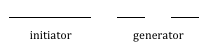Exercises

Iterated Fractals

Using the initiator and generator shown, draw the next two stages of the iterated fractal.

 1.2.3.4.5.6.2. Create a version of the branching tree fractal from example #3 with added randomness.

Fractal Dimension

1. Determine the fractal dimension of the Koch curve.
2. Determine the fractal dimension of the curve generated in exercise #1
3. Determine the fractal dimension of the Sierpinski carpet generated in exercise #5
4. Determine the fractal dimension of the Cantor set generated in exercise #4

Complex Numbers

1. Plot each number in the complex plane:
1. 4
2. –3i
3. $–2+3i$
4. $2 + i$
2. Plot each number in the complex plane:
1. $–2$
2. $4i$
3. $1+2i$
4. $–1–i$
3. Compute:
1. $(2+3i)+(3–4i)$
2. $(3–5i)–(–2–i)$
4. Compute:
1. $(1–i)+(2+4i)$
2. $(–2–3i)–(4–2i)$
5. Multiply:
1. $3\left(2+4i\right)$
2. $(2i)\left(-1-5i\right)$
3. $\left(2-4i\right)\left(1+3i\right)$
6. Multiply:
1. $2\left(-1+3i\right)$
2. $(3i)\left(2-6i\right)$
3. $\left(1-i\right)\left(2+5i\right)$
7. Plot the number $2+3i$. Does multiplying by $1-i$ move the point closer to or further from the origin? Does it rotate the point, and if so which direction?
8. Plot the number $2+3i$. Does multiplying by $0.75+0.5i$ move the point closer to or further from the origin?   Does it rotate the point, and if so which direction?

Recursive Sequences

1. Given the recursive relationship${{z}_{n+1}}=i{{z}_{n}}+1,\quad{{z}_{0}}=2$, generate the next 3 terms of the recursive sequence.
2. Given the recursive relationship ${{z}_{n+1}}=2{{z}_{n}}+i,\quad{{z}_{0}}=3-2i$, generate the next 3 terms of the recursive sequence.
3. Using $c=–0.25$, calculate the first 4 terms of the Mandelbrot sequence.
4. Using $c=1–i$, calculate the first 4 terms of the Mandelbrot sequence.

For a given value of c, the Mandelbrot sequence can be described as escaping (growing large), a attracted (it approaches a fixed value), or periodic (it jumps between several fixed values). A periodic cycle is typically described the number if values it jumps between; a 2-cycle jumps between 2 values, and a 4-cycle jumps between 4 values.

For questions 25 – 30, you’ll want to use a calculator that can compute with complex numbers, or use an online calculator which can compute a Mandelbrot sequence. For each value of c, examine the Mandelbrot sequence and determine if the value appears to be escaping, attracted, or periodic?

1. $c=-0.5+0.25i$.
2. $c=0.25+0.25i$.
3. $c=-1.2$.
4. $c=i$.
5. $c=0.5+0.25i$.
6. $c=-0.5+0.5i$.
7. $c=-0.12+0.75i$.
8. $c=-0.5+0.5i$.

Exploration

The Julia Set for c is another fractal, related to the Mandelbrot set. The Julia Set for c uses the recursive sequence: ${{z}_{n+1}}={{z}_{n}}^{2}+c,\quad{{z}_{0}}=d$, where c is constant for any particular Julia set, and d is the number being tested. A value d is part of the Julia Set for c if the sequence does not grow large.

For example, the Julia Set for -2 would be defined by ${{z}_{n+1}}={{z}_{n}}^{2}-2,\quad{{z}_{0}}=d$. We then pick values for d, and test each to determine if it is part of the Julia Set for -2. If so, we color black the point in the complex plane corresponding with the number d. If not, we can color the point d based on how fast it grows, like we did with the Mandelbrot Set.

For questions 33-34, you will probably want to use the online calculator again.

1. Determine which of these numbers are in the Julia Set at $c=-0.12i+0.75i$
1. a) $0.25i$
2. b) $0.1$
3. c) $0.25+0.25i$
2. Determine which of these numbers are in the Julia Set at
1. a) $0.5i$
2. b) $1$
3. c) $0.5-0.25i$

You can find many images online of various Julia Sets.

1. Explain why no point with initial distance from the origin greater than 2 will be part of the Mandelbrot sequence